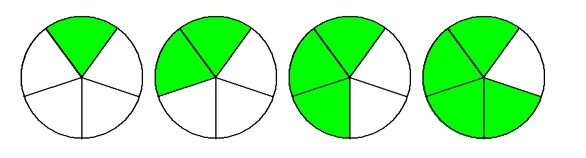# Exam Questions – First Term Examination Mathematics Primary 3 (Basic 3)

Last Updated on May 24, 2021 by Alabi M. S.

MATHEMATICS

FIRST TERM EXAMINATION

CLASS – PRIMARY 3 (BASIC 3)

INSTRUCTION: Answer all the questions.

SECTION A

Answer all the questions in this section.

Choose the correct answer from the options:

1. What is the name of this symbol < ?

[a] greater than

[b] less than

[c] equal to

2. Write 325 in expanded form.

[a] three hundred and twenty five

[b] 3 hundred 2 tens 5 units

[c] three thousand and 5

3. Write 600 is greater than 400 in symbol.

[a] 600 < 400

[b] 600 = 400

[c] 600 > 400

4. Write 134 is lesser than 1483.

[a] 134 > 1483

[b] 134 < 1483

[c] 134 = 1483

5. Excel had 53 mables when he started the game, he lost 15 marbles, How many does he have left?

[a] 49

[b] 38

[c] 80

6. Bolaji collected 610 newspaper for recycling, Neza collected 241 newspaper, How many newspaper were collected altogether?

[a] 851

[b] 900

[c] 2020

7. Write in figure; Five thousand and one hundred.

[a] 50100

[b] 5000100

[c] 5100

8. What is 3/4 of 56?

[a] 42

[b] 40

[c] 36

9. 9 multiply by 9.

[a] 72

[b] 81

[c] 42

10. 40 divided by 4.

[a] 12

[b] 30

[c] 100

11. Subtract 100 from 350.

[a] 220

[b] 210

[c] 250

12. What is the sum of 219 and 378.

[a] 597

[b] 523

[c] 719

13. What is the fraction of the square shaded?[a] 2/6

[b] 3/6

[c] 4/6

14. What is the fraction of the circle shaded?[a] 5/8

[b] 4/8

[c] 3/8

15. What is the value of 3 in 345.

[a] unit

[b] hundred

[c] tens

SECTION B

Attempt all questions

1a. Add 402 to 325.

b. What is the sum of these numbers 345 + 302 + 315

c. Abu is collected 610 newspaper for selling. Peter collected 241 newspaper, How much were they altogether?

d. One hundred and twenty five plus thirty.

2a. Subtract 362 from 612.

b. Find the differences between 572 and 231

c.

H  T   U

6  7   2

– 1  2   6

_____________

_____________

3. Solve these:

a. 1/3 of 15 =

b. 1/5 of 100 =

c. 1/4 of 60 =

d. 1/2 of 20 =

4. Write the following numbers in word:

a. 1259 = ___________________________________________________

b. 1010 = ___________________________________________________

c. 356 =   ___________________________________________________

d. 5,678 = ___________________________________________________

5. What fraction of each shape is shaded:

a.                            b.                          c.                          d.Smart Teachers Plan Lesson Notes - ClassRoomNotes support teachers with hands-on lesson plans/notes, printable and thoughtful teaching resources. @ClassRoomNotes - We always love to hear from you always. Stay connected with your classroom.
error: